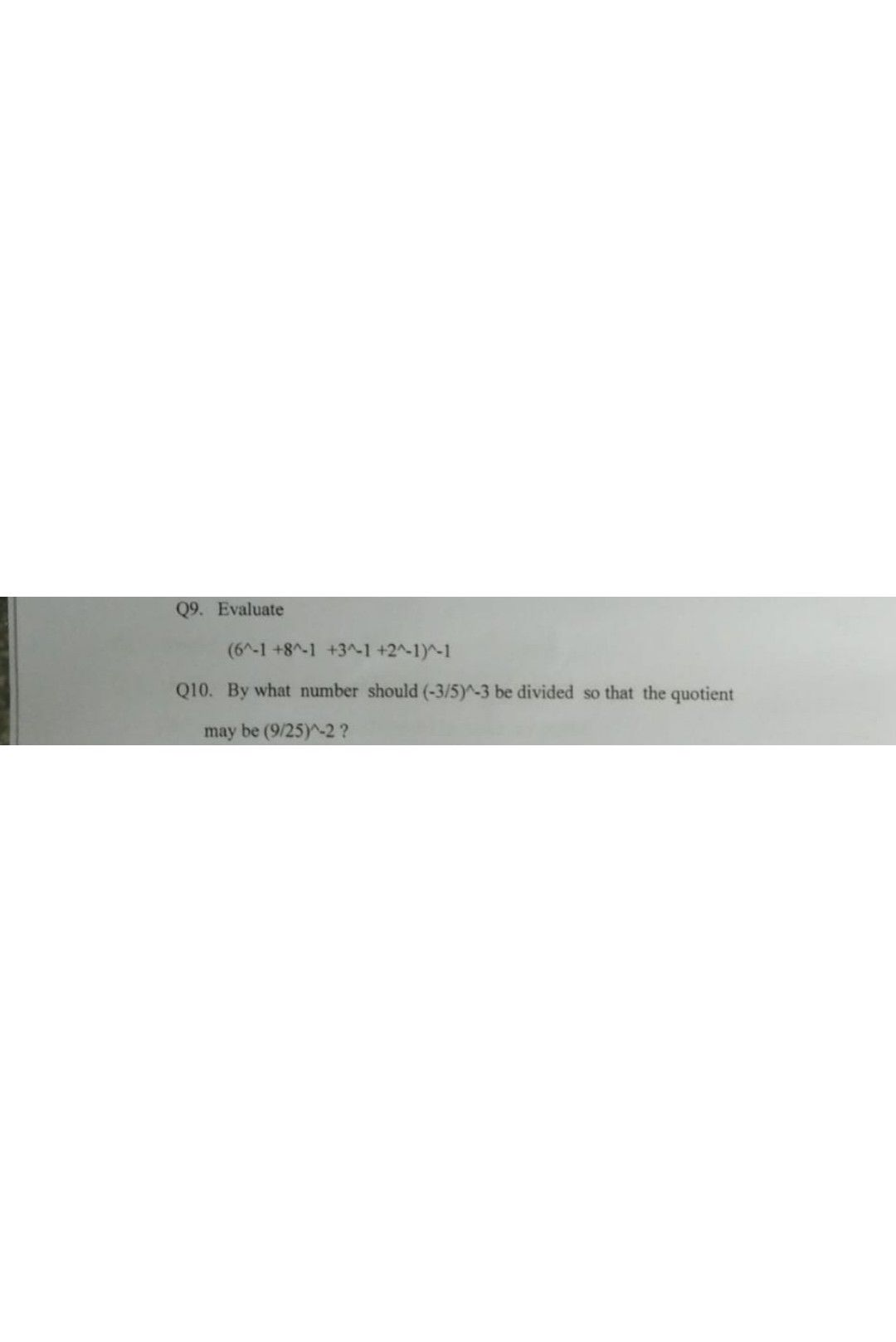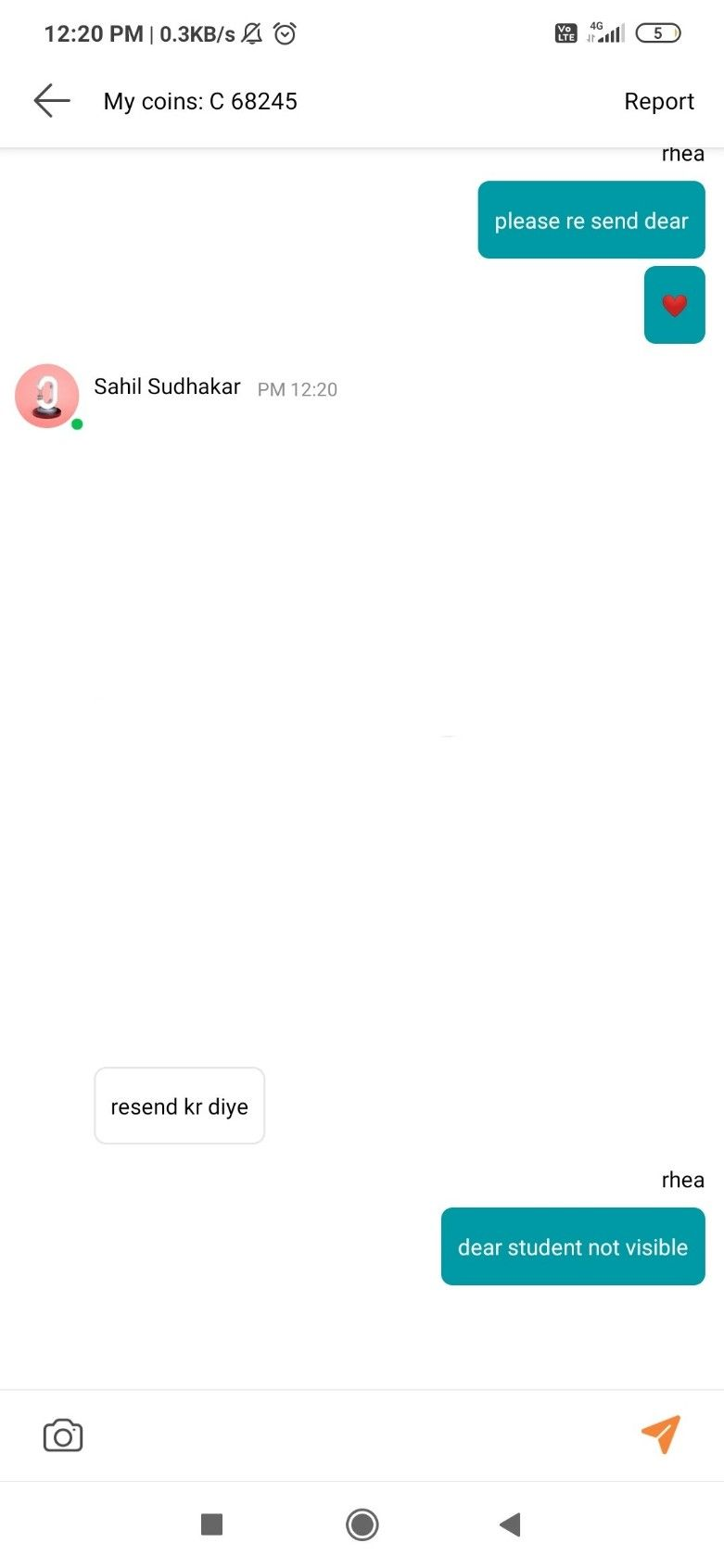Symbol
Problem$09.$ Evaluate $\left(6n.1+8n.1$ $+3n.1+2n-1\right)$ $1$ $010.$ By what number should $\left(.315\right)-3$ be divided so that the quotient may be $\left(925\right).22$
Algebra
Solution❤️Studentresend kr diye